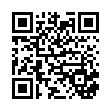# Antenna .pdf

### File information

Original filename: Antenna.pdf

This PDF 1.4 document has been generated by / iText by lowagie.com (r1.02b;p128), and has been sent on pdf-archive.com on 10/05/2014 at 17:09, from IP address 202.51.x.x. The current document download page has been viewed 844 times.
File size: 5.4 MB (132 pages).
Privacy: public file

Antenna.pdf (PDF, 5.4 MB)

### Document preview

POKHARA UNIVERSITY
Level: Bachelor
Semester – Fall
Programme: BE
Course: Electromagnetic Propagation and Antenna

Year
: 2005
Full Marks: 100
Time
: 3hrs.

Candidates are required to give their answers in their own words as far
as practicable.
The figures in the margin indicate full marks.
Attempt all the questions.
1. Find the expression for power radiated by a current element.

15

2. a) What do you mean by the directive gain of an antenna? Show that the
directivity of a half wave dipole is 2.15 dBi.

7

b) Prove that maximum effective area of any antenna is 1.5 λ2 / 4π

8

3. a) What do you mean by parasitic array. Discuss about Yagi-Uda
antenna.

8

b) Find the pattern for an eight-element array obtained by principle of
multiplication of patterns.

7

(FSL) in decibel (dB) and signal-to-noise ratio (S/N) for a receiving
system.

8

b) Consider a link at a frequency f = 14 GHz between a TV satellite in
geostationary orbit and a parabolic receiving antenna of the surface of
the Earth, at a distance d= 36,000 Km from the satellite. The transmit
power is PT = 100 W, and the transmit antenna gain is GT = 40 dB.

7

i. Determine the power density dP/dS (Watt/m2) at the
ii. The TV picture quality is acceptable if the receiver antenna
(assumed lossless) receives a power PR which exceeds a
threshold P0 = 2×10-11 W. What should the antenna area SA
and the antenna gain GA (in dB) to achieve this?
5. a) Define Fresnel reflection coefficient ( Γ) . Assuming earth as lossy
dielectric medium, derive the expression for reflection coefficient
when E-field is in plane of incidence.

7

b) Derive the expression for refractive index of ionosphere and the
maximum usable frequency (MUF).

8

6. a) Consider the case for synchronous satellite relay, where 6 GHz is used
for ground to satellite link and 4 GHz is used for satellite to ground
link. Consider 28 meter diameter ground antenna and 0.28 meter
diameter satellite antenna assuming 67% effective area and height of
the satellite is 36,000 km. Find the following:
i.

10

Basic transmission loss

ii. Maximum directive gain
iii. With ground transmitter power of 12kw, the power
iv. With satellite transmitter power of 1w, the power received
at ground antenna.
b) Draw the block diagram of optical fiber communication. Explain each
block in brief.
7. Write short notes on (Any Two)
a) Horn antenna
b) SID
c) Half power beamwidth
d) Antenna Temperature and signal to Noise ratio.

5

2×5

#### HTML Code

Copy the following HTML code to share your document on a Website or Blog

#### QR Code### Related keywords